# How to Calculate and Solve for Rate at Which Latent Heat is Evolved | Solidification of Metals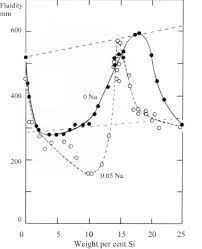The image above represents rate at which latent heat is evolved.

To compute for rate at which latent heat is evolved, three essential parameters are needed and these parameters are Density of Solidifying Metal (ρ’), Latent Heat of Fusion of Metal (Hf) and Mass Flow Rate (dM/dt).

The formula for calculating rate at which latent heat is evolved:

qx = ρ’Hf(dM/dt)

Where:

qx = Rate at Which Latent Heat is Evolved
ρ’ = Density of Solidifying Metal
Hf = Latent Heat of Fusion of Metal
dM/dt = Mass Flow Rate

Let’s solve an example;
Find the rate at which latent heat is evolved when the density of solidifying metal is 8, the latent heat of fusion of metal is 4 and the mass flow rate is 2.

This implies that;

ρ’ = Density of Solidifying Metal = 8
Hf = Latent Heat of Fusion of Metal = 4
dM/dt = Mass Flow Rate = 2

qx = ρ’Hf(dM/dt)
qx = (8)(4)(2)
qx = 64

Therefore, the rate at which latent heat is evolved is 64.

Calculating the Density of Solidifying Metal when the Rate at which Latent Heat is Evolved, the Latent Heat of Fusion of Metal and the Mass Flow Rate is Given.

ρ’ = qx / Hf (dM/dt)

Where;

ρ’ = Density of Solidifying Metal
qx = Rate at Which Latent Heat is Evolved
Hf = Latent Heat of Fusion of Metal
dM/dt = Mass Flow Rate

Let’s solve an example;
Find the density of solidifying metal when the rate at which latent heat is evolved is 25, the latent heat of fusion of metal is 5 and the mass flow rate is 3.

This implies that;

qx = Rate at Which Latent Heat is Evolved = 25
Hf = Latent Heat of Fusion of Metal = 5
dM/dt = Mass Flow Rate = 3

ρ’ = qx / Hf (dM/dt)
ρ’ = 25 / 5 (3)
ρ’ = 25 / 15
ρ’ = 1.67

Therefore, the density of solidifying metal is 1.67.

Calculating the Latent Heat of Fusion of Metal when the Rate at which Latent Heat is Evolved, the Density of Solidifying Metal and the Mass Flow Rate is Given.

Hf = qx / ρ’ (dM/dt)

Where;

Hf = Latent Heat of Fusion of Metal
qx = Rate at Which Latent Heat is Evolved
ρ’ = Density of Solidifying Metal
dM/dt = Mass Flow Rate

Let’s solve an example;
Find the latent heat of fusion of metal when the rate at which latent heat is evolved is 30, the density of solidifying metal is 6 and the mass flow rate is 4.

This implies that;

qx = Rate at Which Latent Heat is Evolved = 30
ρ’ = Density of Solidifying Metal = 6
dM/dt = Mass Flow Rate = 4

Hf = qx / ρ’ (dM/dt)
Hf = 30 / 6 (4)
Hf = 30 / 24
Hf = 1.25

Therefore, the latent heat of fusion of metal is 1.25.

Calculating the Mass Flow Rate when the Rate at which Latent Heat is Evolved, the Density of Solidifying Metal and the Latent Heat of Fusion of Metal is Given.

dM/dt = qx / ρ’Hf

Where;

dM/dt = Mass Flow Rate
qx = Rate at Which Latent Heat is Evolved
ρ’ = Density of Solidifying Metal
Hf = Latent Heat of Fusion of Metal

Let’s solve an example;
Find the mass flow rate when the rate at which latent heat is evolved is 28, the density of solidifying metal is 4 and the latent heat of fusion of metal is 3.

This implies that;

qx = Rate at Which Latent Heat is Evolved = 28
ρ’ = Density of Solidifying Metal = 4
Hf = Latent Heat of Fusion of Metal =3

dM/dt = qx / ρ’Hf
dM/dt = 28 / (4)(3)
dM/dt = 28 / 12
dM/dt = 2.33

Therefore, the mass flow rate is 2.33.

Nickzom Calculator – The Calculator Encyclopedia is capable of calculating the rate at which latent heat is evolved.

To get the answer and workings of the rate at which latent heat is evolved using the Nickzom Calculator – The Calculator Encyclopedia. First, you need to obtain the app.

You can get this app via any of these means:

To get access to the professional version via web, you need to register and subscribe for NGN 2,000 per annum to have utter access to all functionalities.
You can also try the demo version via https://www.nickzom.org/calculator

Apple (Paid) – https://itunes.apple.com/us/app/nickzom-calculator/id1331162702?mt=8
Once, you have obtained the calculator encyclopedia app, proceed to the Calculator Map, then click on Materials and Metallurgical under Engineering.Now, Click on Solidification of Metal under Materials and Metallurgical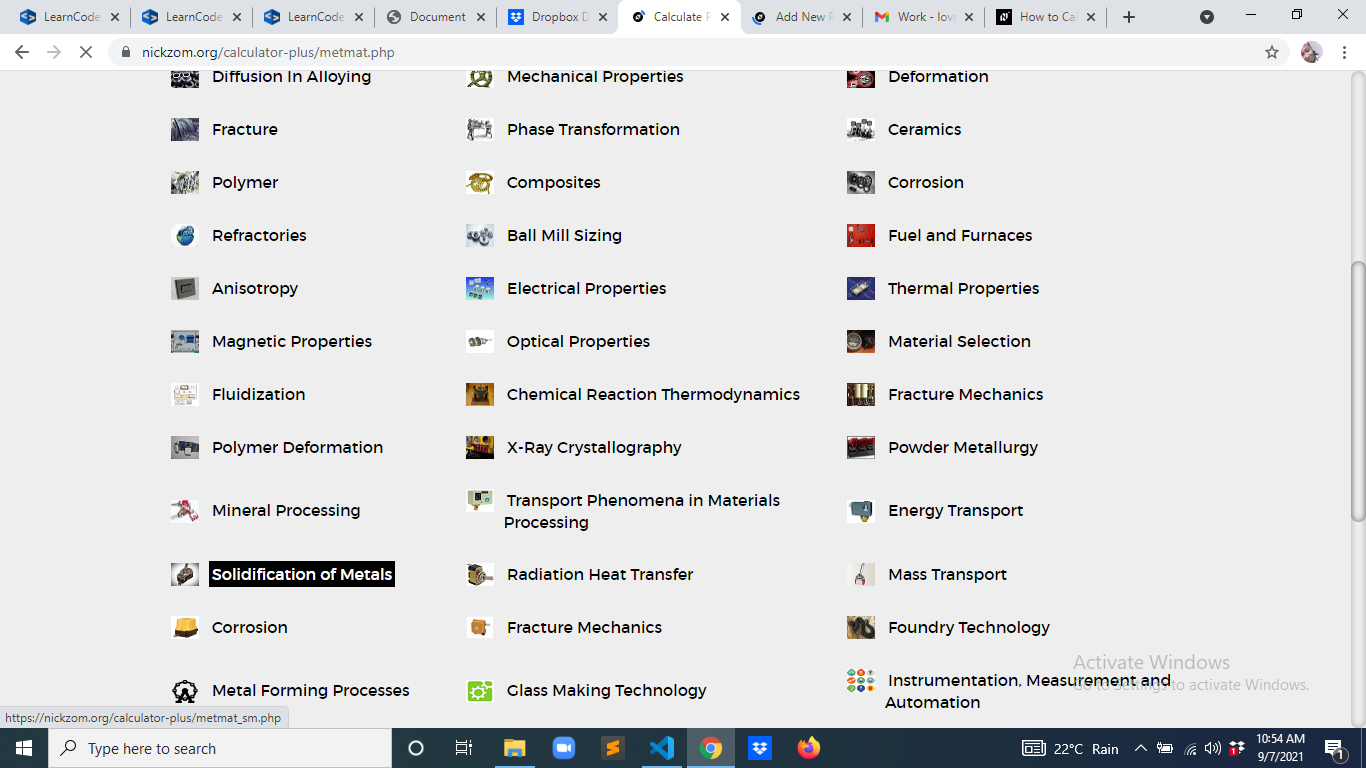Now, Click on Rate at which Latent Heat is Evolved under Solidification of Metal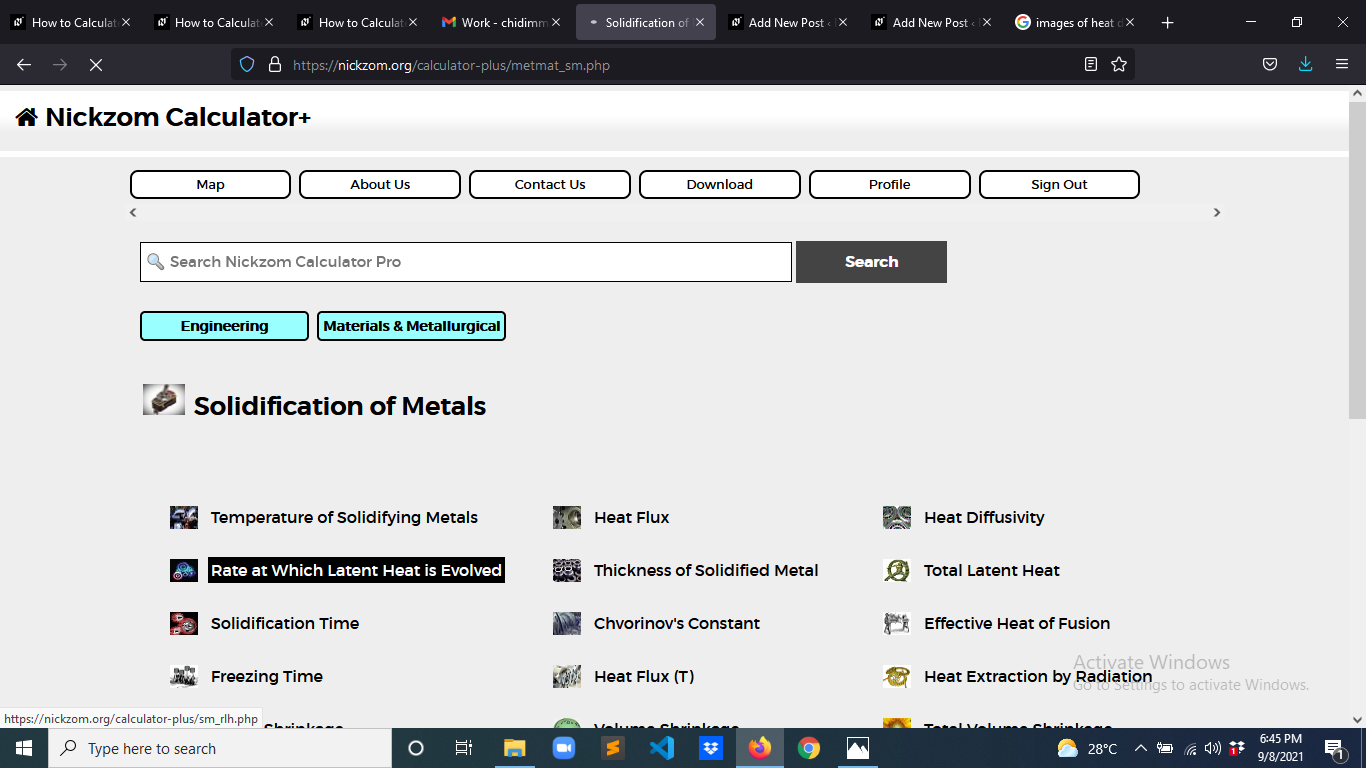The screenshot below displays the page or activity to enter your values, to get the answer for the rate at which latent heat is evolved according to the respective parameter which is the Density of Solidifying Metal (ρ’), Latent Heat of Fusion of Metal (Hf) and Mass Flow Rate (dM/dt).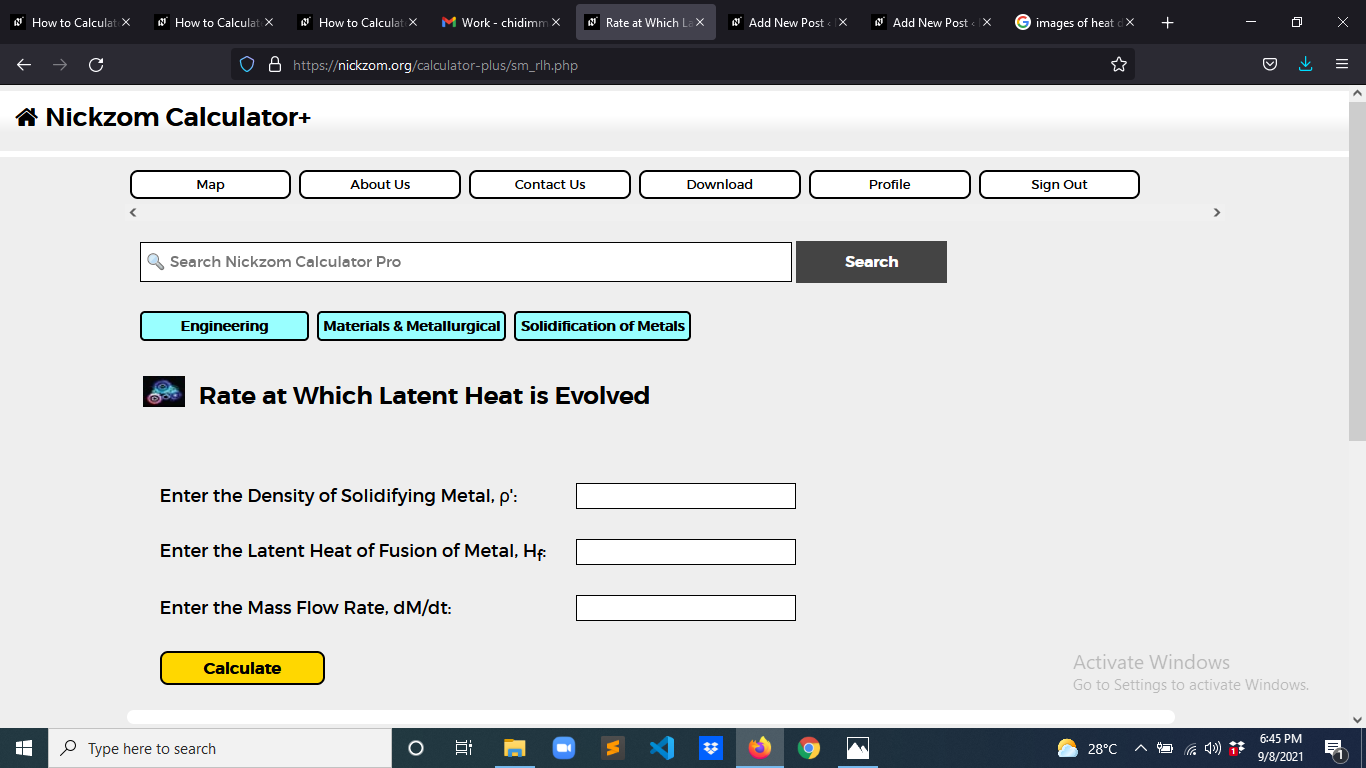Now, enter the values appropriately and accordingly for the parameters as required by the Density of Solidifying Metal (ρ’) is 8, Latent Heat of Fusion of Metal (Hf) is 4 and Mass Flow Rate (dM/dt) is 2.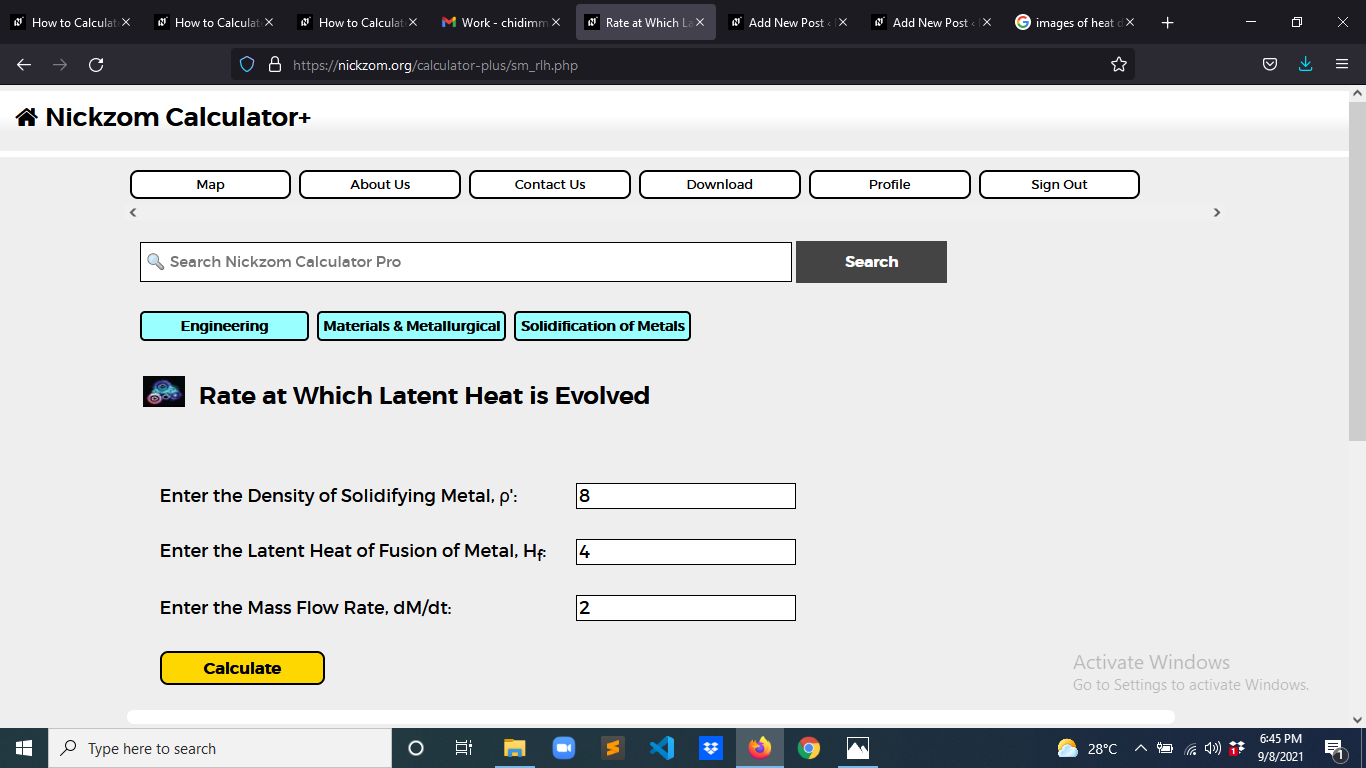Finally, Click on Calculate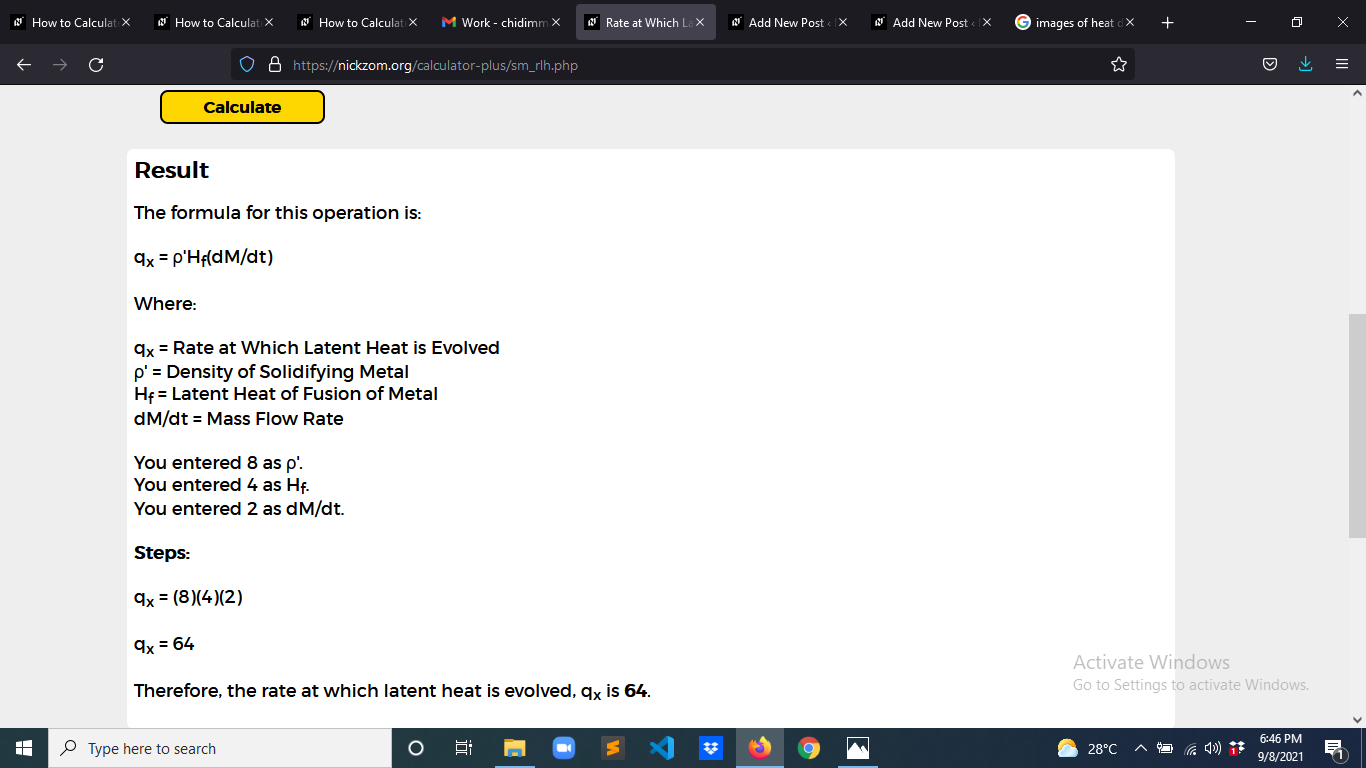As you can see from the screenshot above, Nickzom Calculator– The Calculator Encyclopedia solves for the rate at which latent heat is evolved and presents the formula, workings and steps too.